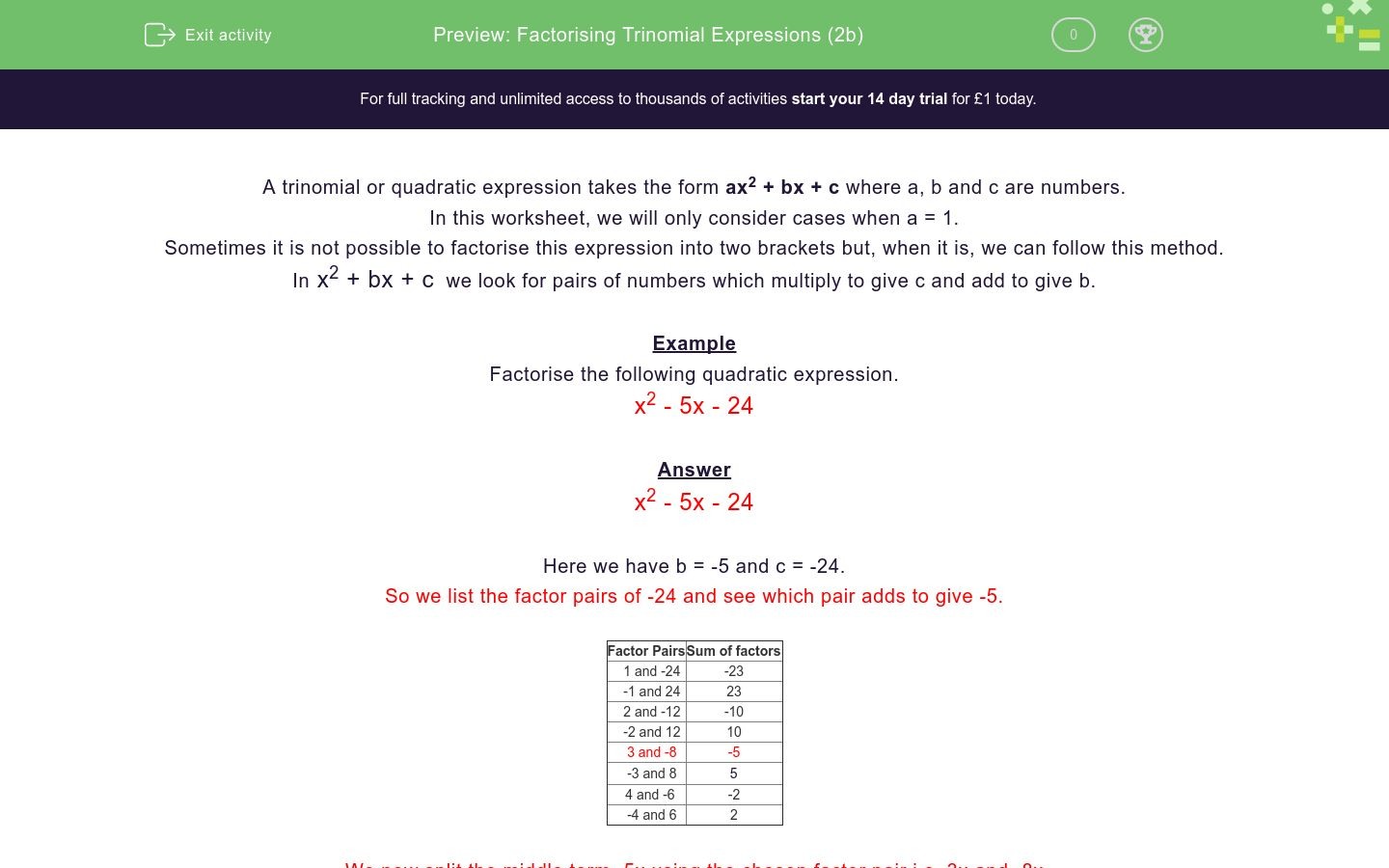# Factorising Trinomial Expressions (2b)

In this worksheet, students factorise simple trinomial expressions.Key stage:  KS 4

Curriculum topic:  Algebra

Curriculum subtopic:  Simplify/Manipulate Algebraic Expressions/Fractions

Difficulty level:### QUESTION 1 of 10

A trinomial or quadratic expression takes the form ax2 + bx + c where a, b and c are numbers.

In this worksheet, we will only consider cases when a = 1.

Sometimes it is not possible to factorise this expression into two brackets but, when it is, we can follow this method.

In x2 + bx + c  we look for pairs of numbers which multiply to give c and add to give b.

Example

Factorise the following quadratic expression.

x2 - 5x - 24

Answer

x2 - 5x - 24

Here we have b = -5 and c = -24.

So we list the factor pairs of -24 and see which pair adds to give -5.

Factor Pairs Sum of factors
1 and -24 -23
-1 and 24 23
2 and -12 -10
-2 and 12 10
3 and -8 -5
-3 and 8

5

4 and -6 -2
-4 and 6 2

We now split the middle term -5x using the chosen factor pair i.e. 3x and -8x

x2 + 3x - 8x - 24

Consider the expression in pairs

x2 + 3x - 8x - 24

Factorise each pair

x (x + 3) - 8 (x + 3)

Combine

(x - 8) (x + 3)

This is the full factorisation

(x - 8) (x + 3)

Factorise the following quadratic expression.

x2 + 6x - 16

(x - 7) (x - 6)

(x + 6) (x - 7)

(x - 8) (x - 2)

(x - 2) (x + 8)

Factorise the following quadratic expression.

x2 - 16x + 28

(x - 7) (x - 4)

(x - 3) (x - 6)

(x - 2) (x - 14)

(x - 2) (x - 9)

Factorise the following quadratic expression.

x2 + 35x - 36

(x + 9) (x - 4)

(x + 3) (x + 12)

(x - 4) (x - 9)

(x - 1) (x + 36)

Factorise the following quadratic expression.

x2 - 10x - 24

(x + 2) (x - 12)

(x - 3) (x + 8)

(x - 3) (x - 8)

(x - 6) (x + 4)

Factorise the following quadratic expression.

x2 - 17x + 16

(x - 4) (x - 4)

(x - 2) (x - 8)

(x + 4) (x - 4)

(x - 16) (x - 1)

Factorise the following quadratic expression.

x2 + 22x + 40

(x + 10) (x + 4)

(x + 2) (x + 20)

(x - 8) (x - 5)

(x - 5) (x + 8)

Factorise the following quadratic expression.

x2 - 15x + 54

(x - 6) (x - 9)

(x - 18) (x - 3)

(x - 3) (x + 18)

(x + 6) (x - 9)

Factorise the following quadratic expression.

x2 - 5x - 24

(x - 6) (x - 4)

(x - 3) (x - 8)

(x - 2) (x - 12)

(x + 3) (x - 8)

Factorise the following quadratic expression.

x2 + 14x - 72

(x - 9) (x + 8)

(x + 6) (x - 12)

(x - 12) (x - 6)

(x + 18) (x - 4)

Factorise the following quadratic expression.

x2 + 12x - 45

(x - 9) (x - 5)

(x - 15) (x - 3)

(x + 15) (x - 3)

(x - 45) (x + 1)

• Question 1

Factorise the following quadratic expression.

x2 + 6x - 16

CORRECT ANSWER
(x - 2) (x + 8)
• Question 2

Factorise the following quadratic expression.

x2 - 16x + 28

CORRECT ANSWER
(x - 2) (x - 14)
• Question 3

Factorise the following quadratic expression.

x2 + 35x - 36

CORRECT ANSWER
(x - 1) (x + 36)
• Question 4

Factorise the following quadratic expression.

x2 - 10x - 24

CORRECT ANSWER
(x + 2) (x - 12)
• Question 5

Factorise the following quadratic expression.

x2 - 17x + 16

CORRECT ANSWER
(x - 16) (x - 1)
• Question 6

Factorise the following quadratic expression.

x2 + 22x + 40

CORRECT ANSWER
(x + 2) (x + 20)
• Question 7

Factorise the following quadratic expression.

x2 - 15x + 54

CORRECT ANSWER
(x - 6) (x - 9)
• Question 8

Factorise the following quadratic expression.

x2 - 5x - 24

CORRECT ANSWER
(x + 3) (x - 8)
• Question 9

Factorise the following quadratic expression.

x2 + 14x - 72

CORRECT ANSWER
(x + 18) (x - 4)
• Question 10

Factorise the following quadratic expression.

x2 + 12x - 45

CORRECT ANSWER
(x + 15) (x - 3)
---- OR ----

Sign up for a £1 trial so you can track and measure your child's progress on this activity.

### What is EdPlace?

We're your National Curriculum aligned online education content provider helping each child succeed in English, maths and science from year 1 to GCSE. With an EdPlace account you’ll be able to track and measure progress, helping each child achieve their best. We build confidence and attainment by personalising each child’s learning at a level that suits them.

Start your £1 trial